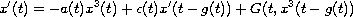Electron. J. Diff. Equ., Vol. 2010(2010), No. 127, pp. 1-8.

### Existence of periodic solutions for neutral nonlinear differential equations with variable delay Deham Hafsia, Djoudi Ahcene

Abstract:
We use a variation of Krasnoselskii fixed point theorem introduced by Burton to show that the nonlinear neutral differential equationhas a periodic solution. Since this equation is nonlinear, the variation of parameters can not be applied directly; we add and subtract a linear term to transform the differential into an equivalent integral equation suitable for applying a fixed point theorem. Our result is illustrated with an example.

Submitted April 15, 2010. Published September 7, 2010.
Math Subject Classifications: 34K20, 45J05, 45D05.
Key Words: Periodic solution; nonlinear neutral differential equation; large contraction, integral equation.

Show me the PDF file (199 KB), TEX file, and other files for this article.Deham Hafsia Department of Mathematics, Faculty of Sciences University of Annaba, P.O. Box 12 Annaba, Algeria email: deh_71@yahoo.fr Djoudi Ahcéne Department of Mathematics, Faculty of Sciences University of Annaba, P.O. Box 12 Annaba, Algeria email: adjoudi@yahoo.com